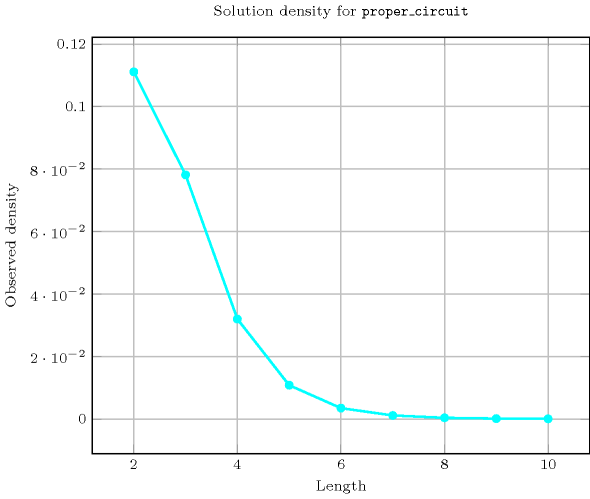## 5.328. proper_circuit

Origin
Constraint

$\mathrm{𝚙𝚛𝚘𝚙𝚎𝚛}_\mathrm{𝚌𝚒𝚛𝚌𝚞𝚒𝚝}\left(\mathrm{𝙽𝙾𝙳𝙴𝚂}\right)$

Synonym

$\mathrm{𝚌𝚒𝚛𝚌𝚞𝚒𝚝}$.

Argument
 $\mathrm{𝙽𝙾𝙳𝙴𝚂}$ $\mathrm{𝚌𝚘𝚕𝚕𝚎𝚌𝚝𝚒𝚘𝚗}\left(\mathrm{𝚒𝚗𝚍𝚎𝚡}-\mathrm{𝚒𝚗𝚝},\mathrm{𝚜𝚞𝚌𝚌}-\mathrm{𝚍𝚟𝚊𝚛}\right)$
Restrictions
 $|\mathrm{𝙽𝙾𝙳𝙴𝚂}|>1$ $\mathrm{𝚛𝚎𝚚𝚞𝚒𝚛𝚎𝚍}$$\left(\mathrm{𝙽𝙾𝙳𝙴𝚂},\left[\mathrm{𝚒𝚗𝚍𝚎𝚡},\mathrm{𝚜𝚞𝚌𝚌}\right]\right)$ $\mathrm{𝙽𝙾𝙳𝙴𝚂}.\mathrm{𝚒𝚗𝚍𝚎𝚡}\ge 1$ $\mathrm{𝙽𝙾𝙳𝙴𝚂}.\mathrm{𝚒𝚗𝚍𝚎𝚡}\le |\mathrm{𝙽𝙾𝙳𝙴𝚂}|$ $\mathrm{𝚍𝚒𝚜𝚝𝚒𝚗𝚌𝚝}$$\left(\mathrm{𝙽𝙾𝙳𝙴𝚂},\mathrm{𝚒𝚗𝚍𝚎𝚡}\right)$ $\mathrm{𝙽𝙾𝙳𝙴𝚂}.\mathrm{𝚜𝚞𝚌𝚌}\ge 1$ $\mathrm{𝙽𝙾𝙳𝙴𝚂}.\mathrm{𝚜𝚞𝚌𝚌}\le |\mathrm{𝙽𝙾𝙳𝙴𝚂}|$
Purpose

Enforce to cover a digraph $G$ described by the $\mathrm{𝙽𝙾𝙳𝙴𝚂}$ collection with one circuit visiting once a subset of the vertices of $G$.

Example
$\left(\begin{array}{c}〈\begin{array}{cc}\mathrm{𝚒𝚗𝚍𝚎𝚡}-1\hfill & \mathrm{𝚜𝚞𝚌𝚌}-2,\hfill \\ \mathrm{𝚒𝚗𝚍𝚎𝚡}-2\hfill & \mathrm{𝚜𝚞𝚌𝚌}-3,\hfill \\ \mathrm{𝚒𝚗𝚍𝚎𝚡}-3\hfill & \mathrm{𝚜𝚞𝚌𝚌}-1,\hfill \\ \mathrm{𝚒𝚗𝚍𝚎𝚡}-4\hfill & \mathrm{𝚜𝚞𝚌𝚌}-4\hfill \end{array}〉\hfill \end{array}\right)$

The $\mathrm{𝚙𝚛𝚘𝚙𝚎𝚛}_\mathrm{𝚌𝚒𝚛𝚌𝚞𝚒𝚝}$ constraint holds since its $\mathrm{𝙽𝙾𝙳𝙴𝚂}$ argument depicts the following circuit visiting successively the vertices 1, 2, 3 and 1 (i.e., node 4 is not visited).

Typical
$|\mathrm{𝙽𝙾𝙳𝙴𝚂}|>2$
Symmetry

Items of $\mathrm{𝙽𝙾𝙳𝙴𝚂}$ are permutable.

Counting
 Length ($n$) 2 3 4 5 6 7 8 9 10 Solutions 1 5 20 84 409 2365 16064 125664 1112073

Number of solutions for $\mathrm{𝚙𝚛𝚘𝚙𝚎𝚛}_\mathrm{𝚌𝚒𝚛𝚌𝚞𝚒𝚝}$: domains $0..n$